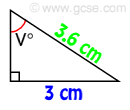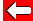# Inverse Sin

Equally important, knowing a ratio between sides, we can use calculators to give us the unknown angle. Easy life!By definition, sinV° = opposite ÷ hypotenuse = 3 ÷ 3·6 = 0·833

We can find V° by telling our calculator that 0·833 is the sin of V°, and it will tell us V if we use inverse-sin. Try this:

[inv] [sin]  [·]    [=]

Some calculators have a second-function button [2nd F] instead. Remember also that older ones will require the number to be put in first. Either way, you should be given the answer:

56.4° or close enough!Go back a pageMaths MenuGo to next page